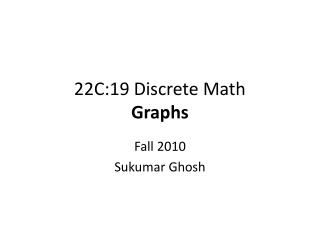DownloadDownload Presentation22C:19 Discrete Math Graphs

# 22C:19 Discrete Math Graphs

Download Presentation## 22C:19 Discrete Math Graphs

- - - - - - - - - - - - - - - - - - - - - - - - - - - E N D - - - - - - - - - - - - - - - - - - - - - - - - - - -
##### Presentation Transcript

1. 22C:19 Discrete MathGraphs Fall 2010 Sukumar Ghosh

2. Seven Bridges of K⍥nigsberg Is it possible to walk along a route that cross each bridge exactly once?

3. Seven Bridges of K⍥nigsberg

4. A Graph

5. What is a Graph A graph G = (V, E) consists of V, a nonempty set of vertices (or nodes) and E, a set of edges. Each edge connects a pair of nodes that are called its endpoints. Graphs are widely used to model various systems in the real world

6. Back to the Bridges of K⍥nigsberg

7. Euler’s solution

8. Euler path

9. Simple graph

10. Types of graph

11. Definitions At most one edge between a pair of nodes Multiple edges between some pair of nodes Simple graphs vs. multi-graphs Undirected vs. directed graphs (digraphs) Each edge between a pair of nodes is directed, and represents an ordered pair

12. More examples of graphs Each node denotes an actor or an actress, and each edge between P and Q denotes that P, Q worked together in some movie Hollywood graph Web graphs Each node denotes a web page, and each edge from page P to Q Q denotes a link on page P pointing to page Q. It is a directed graph

13. Application: Exam scheduling

14. Problems in a computer network

15. Application: graph orientation

16. Vertex degree

17. Degree sequence

18. Handshaking theorem

19. Handshaking theorem

20. A theorem THEOREM. An undirected graph has even number of vertices of odd degree. Can you prove this? It should follow from the handshaking theorem.

21. Review of basic definitions

22. Review of basic definitions

23. Types of graphs

24. Types of graphs The n-cube graph n=3 Complete graph: All vertices are adjacent Wheel graph

25. Types of graphs Bipartite graph A simple graph is called bipartite if its vertex set V can be partitioned into two disjoint subsets V1 and V2, such that every edge in the graph connects a vertex in V1 to a vertex in V2. Can always be colored using two colors.

26. Subgraphs

27. Computer representation of graphs ADJACENCY MATRIX (taken from Wolfram Mathworld)

28. Computer representation of graphs ADJACENCY LIST 1 2 4 3 Can be represented as a linked list

29. Graph isomorphism

30. Graph isomorphism

31. Graph isomorphism

32. Connectivity • An undirected graph is connected if there is a path between • every pair of distinct vertices of the graph. • A connected componentis the maximal connected subgraph • of the given graph.

33. Connectivity in directed graphs A directed graph is stronglyconnected if there is a path from any vertex a to any other vertex b of the graph. A directed graph is weaklyconnected if there is a path between any two vertices of the underlying undirected graph.

34. More definitions Vertex cover is a famous problem in graph theory

35. Euler path vs. Hamiltonian path Hamiltonian path = A path that passes through every vertex exactly once. A closed path is a Hamiltonian circuit or cycle. Euler path = A path that includes every edge exactly once. A closed path is a Euler circuit or cycle. We have reviewed Euler path in the 7-bridges of Konigsberg Problem.

36. Hamiltonian path 1 2 4 5 3 Does the above graph have a Hamiltonian cycle? No! Hamiltonian circuit/cycle colored red

37. Traveling Salesman Problem (TSP) A traveling salesman wants to visit each of n cities exactly once, and then return to the starting point. In which order should he visit the cities to travel the minimum total distance? TSP = Computing the minimum cost Hamiltonian circuit. TSP is an extremely complex problem to solve (NP-complete) An optimal TSP tour through Germany’s largest cities (Source: Wikipedia)

38. Planar Graph A planar graph is one that can be embedded in the plane, i.e., it can be drawn on the plane in such a way that its edges do not intersect except only at their endpoints. planar K4 Butterfly planar Non-planar Non-planar K5 K3,3